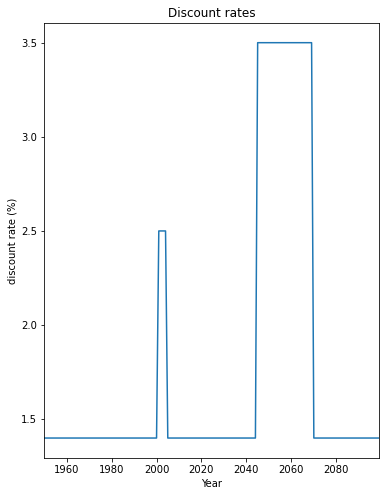# DiscRates class#

Discount rates are used to calculate the net present value of any future or past value. They are thus used to compare amounts paid (costs) and received (benefits) in different years. A project is economically viable (attractive), if the net present value of benefits exceeds the net present value of costs - a cost-benefit ratio < 1.

There are several important implications that come along with discount rates. Namely, that higher discount rates lead to smaller net present values of future impacts (costs). As a consequence of that, climate action and mitigation measures can be postboned. In the literature higher discount rates are typically justified by the expectation of continued exponential growth of the economy. The most widley used interest rate in climate change economics is 1.4% as propsed by the Stern Review (2006). Neoliberal economists around Nordhaus (2007) claim that rates should be higher, around 4.3%. Environmental economists argue that future costs shouldn’t be discounted at all. This is especially true for non-monetary variables such as ecosystems or human lifes, where no price tag should be applied out of ethical reasons. This discussion has a long history, reaching back to the 18th century: “Some things have a price, or relative worth, while other things have a dignity, or inner worth” (Kant, 1785).

This class contains the discount rates for every year and discounts given values. Its attributes are:

• years (np.array): years

• rates (np.array): discount rates for each year (between 0 and 1)

For a complete class documentation, refer to the Python modules docs: climada.entity.disc_rates.base.DiscRates

An example of use - we define discount rates and apply them on a coastal protection scheme which initially costs 100 mn. USD plus 75’000 USD mainteance each year, starting after 10 years. Net present value of the project can be calculated as displayed:

%matplotlib inline
import numpy as np

# define discount rates
years = np.arange(1950, 2100)
rates = np.ones(years.size) * 0.014
rates[51:55] = 0.025
rates[95:120] = 0.035
disc = DiscRates(years=years, rates=rates)
disc.plot()

# Compute net present value between present year and future year.
ini_year = 2019
end_year = 2050
val_years = np.zeros(end_year-ini_year+1)
val_years = 100000000 # initial investment
val_years[10:] = 75000 # maintenance from 10th year
npv = disc.net_present_value(ini_year, end_year, val_years)
print('net present value: {:.5e}'.format(npv))

net present value: 1.01231e+08## Read discount rates of an Excel file

Discount rates defined in an excel file following the template provided in sheet discount of climada_python/climada/data/system/entity_template.xlsx can be ingested directly using the method from_excel().

from climada.entity import DiscRates

# Fill DataFrame from Excel file
file_name = ENT_TEMPLATE_XLS # provide absolute path of the excel file
disc = DiscRates.from_excel(file_name)
disc.plot();

Read file: /Users/ckropf/climada/data/entity_template.xlsx

<AxesSubplot:title={'center':'Discount rates'}, xlabel='Year', ylabel='discount rate (%)'>## Write discount rates#

Users may write the discounts in Excel format using write_excel() method write_excel().

from climada.entity import DiscRates

# Fill DataFrame from Excel file
file_name = ENT_TEMPLATE_XLS # provide absolute path of the excel file
disc = DiscRates.from_excel(file_name)

# write file
disc.write_excel('results/tutorial_disc.xlsx')


Pickle can always be used as well:

from climada.util.save import save
# this generates a results folder in the current path and stores the output there
save('tutorial_disc.p', disc)